# SBI PO Reasoning Questions 2019 (Day-45) High Level-New Pattern

Dear Aspirants, Our IBPS Guide team is providing new series of Reasoning Questions for SBI PO 2019 so the aspirants can practice it on a daily basis. These questions are framed by our skilled experts after understanding your needs thoroughly. Aspirants can practice these new series questions daily to familiarize with the exact exam pattern and make your preparation effective.

[WpProQuiz 6693]

Directions (1-5): Refer to the following data and answer the questions that follow:

A numerical machine accepts two values X and Y. Then it updates these values as X = X*Y and Y = Y+1 in every step. The machine stops at X = N.

1) For X = 3, Y = 2 and N = 360. How many steps are performed before the machine stops (Consider X=3; Y=2 as Input)?

(a) 2

(b) 3

(c) 4

(d) 5

(e) None of these

2) For X = 3, Y = 2 and N = 360. What is the final value of Y (Consider X=3; Y=2 as Input)?

(a) 4

(b) 5

(c) 6

(d) 20

(e) None of these

3) If X = 4 and Y = 5, what should be the value of Y such that final value of N is 840?

(a) 5

(b) 6

(c) 8

(d) 10

(e) None of these

4) If X = 2 and Y = 3, what should be the value of N such that final value of Y is 7?

(a) 300

(b) 360

(c) 860

(d) 720

(e) None of these

5) In the given three statements, find the one in which the given N is true with respect to X and Y in Step-3?

I) X= 3; Y=2; N= 72

II) X=2; Y=3; N= 120

III) X=4;  Y=5;         N= 720

(a) Only I is true

(b) Both I and II are true

(c) Only II is true

(d) Both I and III are true

(e) All I, II, and III are true

Directions (6-10): Study the following information carefully and answer the below questions.

In a grocery shop twelve boxes viz. – B, D, E, G, H, K, M, P, R, S, V and W are kept in four columns as shown in below figure. Each box is marked from BI to BXII. Weight (kg) of each box is also different viz. 2, 3, 4, 7, 8, 9, 13, 14, 16, 17, 19 & 21kg. Boxes adjacent to each other mean either east-west or north-south. All the information is not necessary in same order.

The box BI is kept two places below the box whose weight is 16kg and is kept immediate south-west of the box BXII. The box H is kept adjacent to the box BVI and is immediate west of the box B. The box R is kept only with the box BVII in the same column. Weight of the box BVII is neither odd number nor perfect square. The box BVII is kept immediate south-east of the box D. The box BXI is kept two places away from the box D, whose weight is neither a prime number nor perfect square. Weight of the box M is 2kg and is neither kept adjacent to the box R nor kept adjacent to the box BIX. The box E is kept immediate south-west of the box whose weight is 8kg, which is kept immediate west of the box BIII. The box whose weight is 2kg is kept immediate north-east of the box S, whose weight is neither 9kg nor kept adjacent to the box whose weight is 13kg. The box BXII is kept two places away from the box whose weight is 13kg. The box P is neither kept adjacent to the box D nor adjacent to the box BIII. Weight of the box P is 14kg and is kept immediate north-west of the box whose weight is 9kg. The box BVI is neither kept adjacent to the box BIII nor kept adjacent to the box P. The box G is neither kept adjacent to the box B nor its weight is 16kg. The box BX is kept at any place above the box W, whose weight is 17kg. The box V is kept exactly between the box BIV and the box G, whose weight is 19kg. The box BV and the box whose weight is 4kg are kept together. Two boxes are kept between the box BII and the box whose weight is 7kg.

6) Which of the following boxes are kept together in the column D?

a)  BX, 13kg & BVI

b) K, BIII & 17kg

c) 8kg, B & BX

d) BXI, 13kg & 17kg

e) None of these

7) What is the position of the box whose weight is 14kg with respect to the box BVIII?

a)  Second to west

b) Immediate south

c) Second to north

d) Immediate south-west

e) None of these

8) What is the weight of the box marked BVI?

a)  4kg

b) 21kg

c) 8kg

d) 9kg

e) None of these

9) In which of the following combination the 3rd box doesn’t kept exactly between 1st and 2nd box?

a)  BVII – 14kg – M

b) BXI – W – BVI

c) BVII – BIX – 2kg

d) BII – P – BIX

e) 17kg – BX – BIII

10) What is the weight of the box marked BX?

a)  3 kg

b) 21kg

c) 8kg

d) 9kg

e) None of these

Direction (1-5) :

In order to solve this set of questions you need to build a table showing how the values of X and Y move in every iteration.

Value of X Value of Y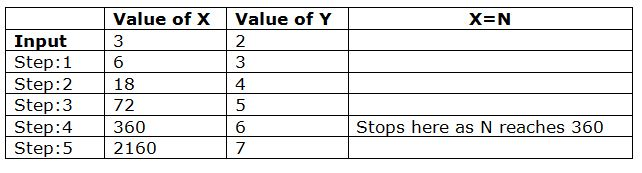Value of X Value of Y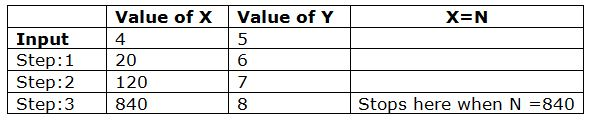Value of X Value of Y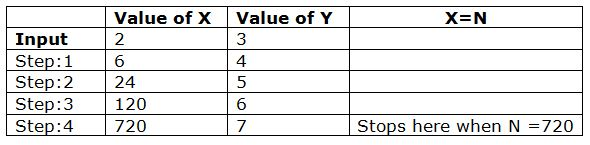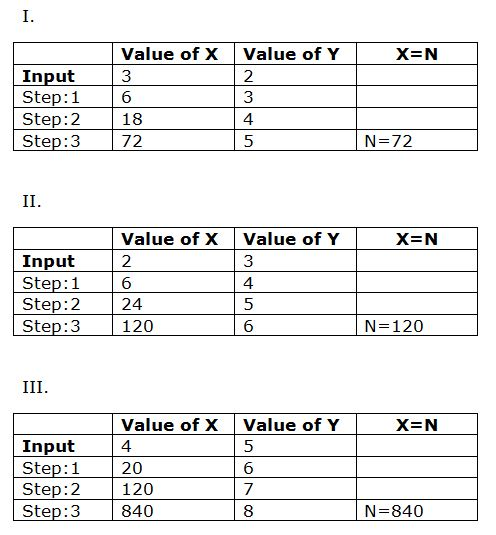Direction (6-10) :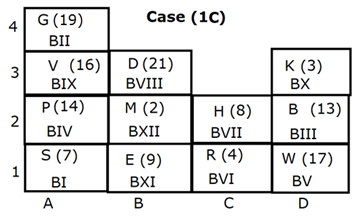We have:

• The box R is kept only with the box marked BVII in the same column, that means the box R must be kept in column C.
• Weight of the box marked BVII is neither odd number nor perfect square.
• The box marked BVII is kept immediate south-east of the box D.
• The box which is marked BXI is kept two places away from the box D, whose weight is neither a prime number nor perfect square, that means in case (1) the box R is kept at 1C and the box marked BXI is kept at 1B, in case (2) the box R is kept at 2C and the box marked BXI is kept at 2D.
• The box whose weight is 2kg is kept immediate north-east of the box S, whose weight is neither 9kg nor kept adjacent to the box whose weight is 13kg.
• Weight of the box M is 2kg and is neither kept adjacent to the box R nor kept adjacent to the box marked BIX, that means in case (1a) the box M is kept at 2B, in case (1b) M is kept at 3D, in case (2) M is kept at 3B.

Based on above given information we have: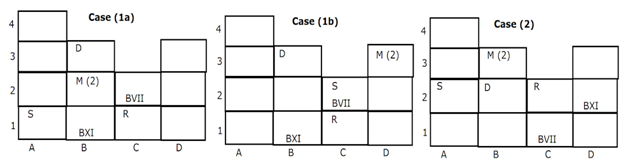Again, we have:

• The box marked BI is kept two places below the box whose weight is 16kg and is kept immediate south-west of the box marked BXII, that means in case (1a), case (1b) & case (2a) the box BI is kept at 2A, in case (1c), case (1d) & case (2b) the box marked BI is kept at 1A.
• The box marked BXII is kept two places away from the box whose weight is 13kg, since the box S is not kept adjacent to the box whose weight is 13kg, thus in case (1b) & case (2a) the box whose weight is 13kg is kept at 1B, in case (1c) & case (2b) the box whose weight is 13kg is kept at 2D and case (1a) & case (1d) are not valid.
• The box E is kept immediate south-west of the box whose weight is 8kg, which is kept immediate west of the box marked BIII, that means in case (1b) & case (1c) the box E is kept at 1B, in case (2a) & case (2b) the box E is kept at 1A.
• The box P is neither kept adjacent to the box D nor adjacent to the box marked BIII.
• Weight of the box P is 14kg and is kept immediate north-west of the box whose weight is 9kg, since weight of the box D and the box marked BVII is not a perfect square, that means in case (1c) the box P is kept at 2A and case (1b), case (2a) & case (2b) are not valid.

Based on above given information we have: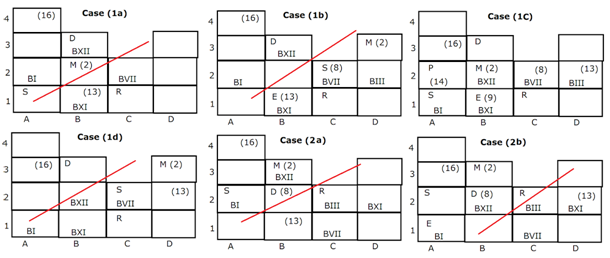Case (1a) & case (1d) are not valid as the box marked BXII is kept two places away from the box whose weight is 13kg, case (1b), case (2a) & case (2b) are not valid as Weight of the box P is 14kg and is kept immediate north-west of the box whose weight is 9kg.

Again, we have:

• The box marked BVI is neither kept adjacent to the box marked BIII nor kept adjacent to the box P.
• The box H is kept adjacent to the box marked BVI and is immediate west of the box B, that means the box marked BVI is kept at 1C and weight of the box B is 13kg.
• The box G is neither kept adjacent to the box B nor its weight is 16kg.
• The box marked V is kept exactly between the box marked BIV and the box G, whose weight is 19kg, that means the box G is kept at 4A.
• The box marked BX is kept at any place above the box W, whose weight is 17kg, that means the box W is kept at 1D and the box marked BX is kept at 3D.
• As, only remaining box is K, thus box K is marked BX.
• The box marked BV and the box whose weight is 4kg are kept together, that means weight of the box R is 4kg.
• Since, only remaining weights are 7kg, 21kg and 3kg, as weight of the box D is not a prime number, thus weight of the box D is 21kg.
• Two boxes are kept between the box marked BII and the box whose weight is 7kg, that means weight of the box S is 7kg.
• Since, the box marked BIX is not kept adjacent to the box M, thus box V is marked BIX.

Based on above given information we have final arrangement: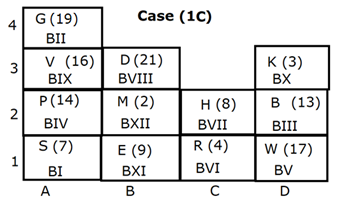Clearly, the boxes K, BIII & 17kg are kept together in column D.

Hence, option B is correct choice.

Clearly, the box whose weight is 14kg is kept immediate south-west of the box BVIII.

Hence, option D is correct choice.

Clearly, weight of the box marked BVI is 4kg.

Hence, option A is correct choice.

Clearly, combination C is correct choice.

Hence, option C is correct choice.